Hits: 3

# Insertion in a Red-Black Tree

#### In this tutorial, you will learn how a new node can be inserted into a red-black tree is. Also, you will find working examples of insertions performed on a red-black tree in C, C++, Java and Python.

Red-Black tree is a self-balancing binary search tree in which each node contains an extra bit for denoting the color of the node, either red or black.

Before reading this article, please refer to the article on red-black tree.

While inserting a new node, the new node is always inserted as a RED node. After insertion of a new node, if the tree is violating the properties of the red-black tree then, we do the following operations.

1. Recolor
2. Rotation

## Algorithm to Insert a New Node

Following steps are followed for inserting a new element into a red-black tree:

1. The `newNode` be:New node
2. Let y be the leaf (ie. `NIL`) and `x` be the root of the tree. The new node is inserted in the following tree.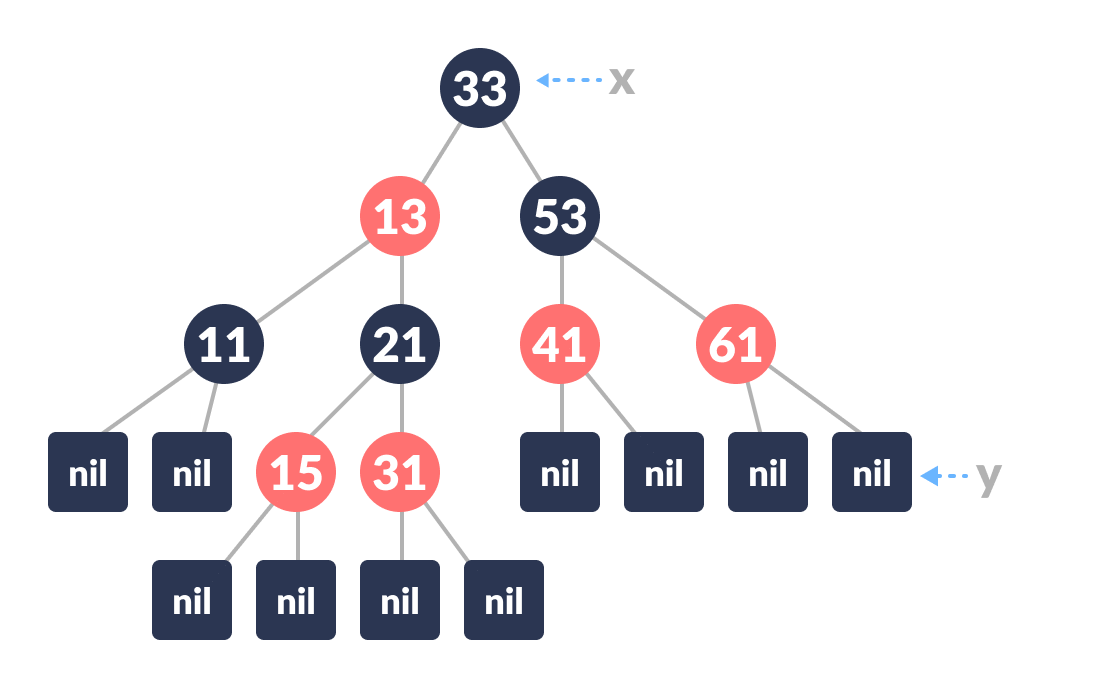Initial tree
3. Check if the tree is empty (ie. whether `x` is `NIL`). If yes, insert `newNode` as a root node and color it black.
4. Else, repeat steps following steps until leaf (`NIL`) is reached.
1. Compare `newKey` with `rootKey`.
2. If `newKey` is greater than `rootKey`, traverse through the right subtree.
3. Else traverse through the left subtree.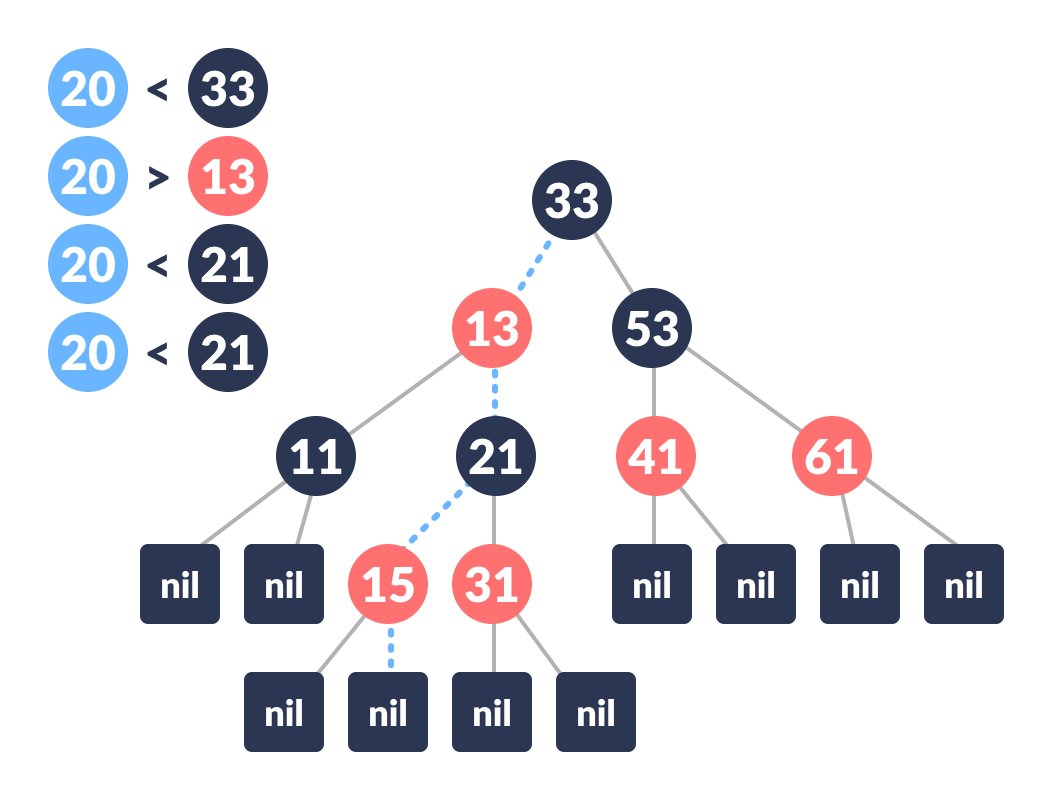Path leading to the node where newNode is to be inserted
5. Assign the parent of the leaf as parent of `newNode`.
6. If `leafKey` is greater than `newKey`, make `newNode` as `rightChild`.
7. Else, make `newNode` as `leftChild`.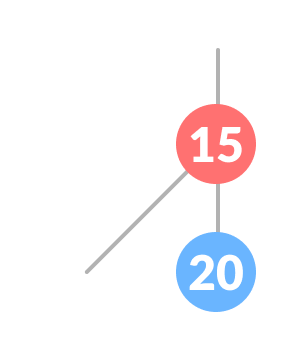New node inserted
8. Assign `NULL` to the left and `rightChild` of `newNode`.
9. Assign RED color to `newNode`.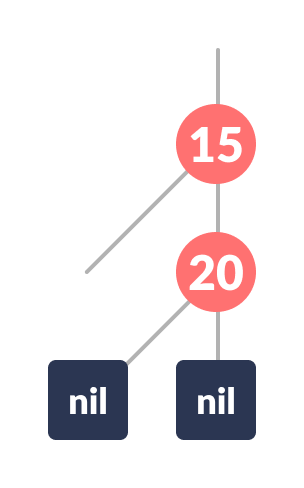Set the color of the newNode red and assign null to the children
10. Call InsertFix-algorithm to maintain the property of red-black tree if violated.

Why newly inserted nodes are always red in a red-black tree?

This is because inserting a red node does not violate the depth property of a red-black tree.

If you attach a red node to a red node, then the rule is violated but it is easier to fix this problem than the problem introduced by violating the depth property.

## Algorithm to Maintain Red-Black Property After Insertion

This algorithm is used for maintaining the property of a red-black tree if insertion of a newNode violates this property.

1. Do the following until the parent of `newNode` `p` is RED.
2. If `p` is the left child of `grandParent` `gP` of `newNode`, do the following.
Case-I:

1. If the color of the right child of `gP` of `newNode` is RED, set the color of both the children of `gP` as BLACK and the color of `gP` as RED.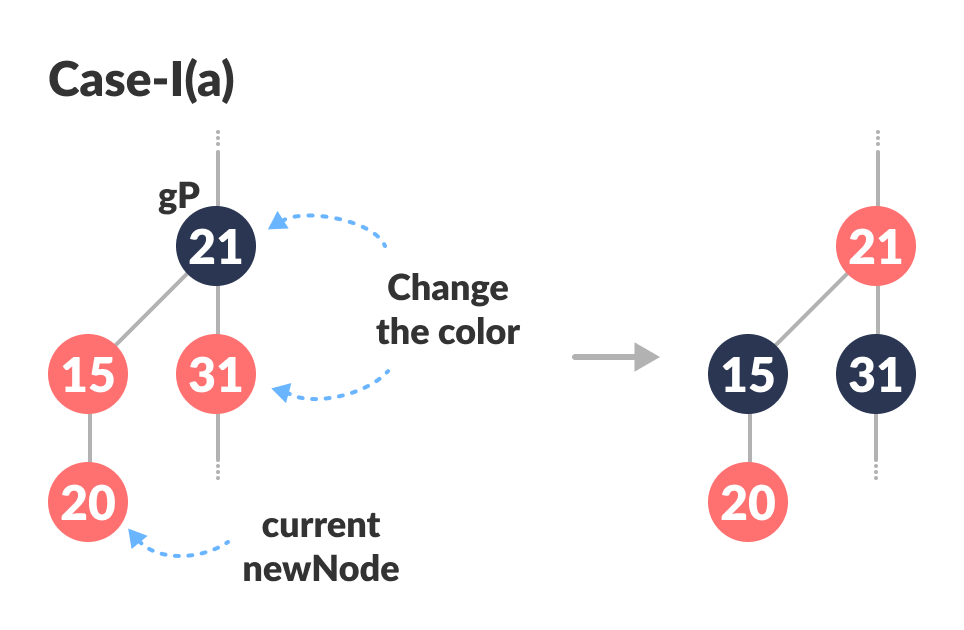Color change
2. Assign `gP` to `newNode`.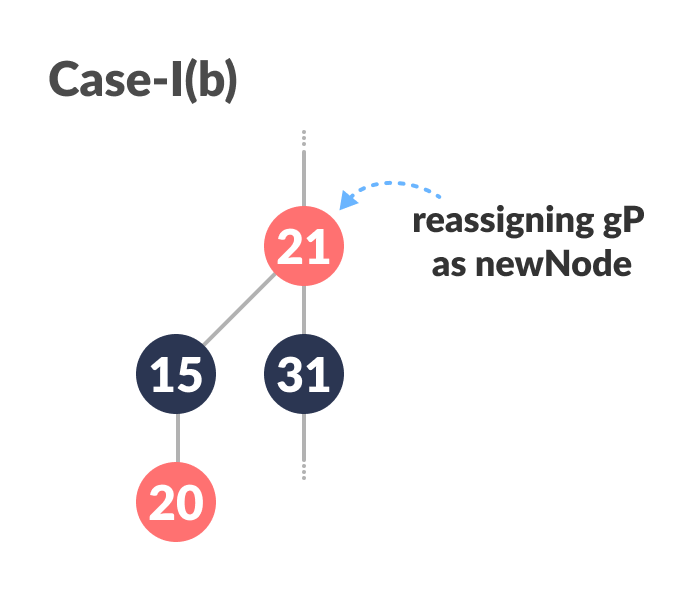Reassigning newNode

Case-II:

3. (Before moving on to this step, while loop is checked. If conditions are not satisfied, it the loop is broken.)
Else if `newNode` is the right child of `p` then, assign `p` to `newNode`.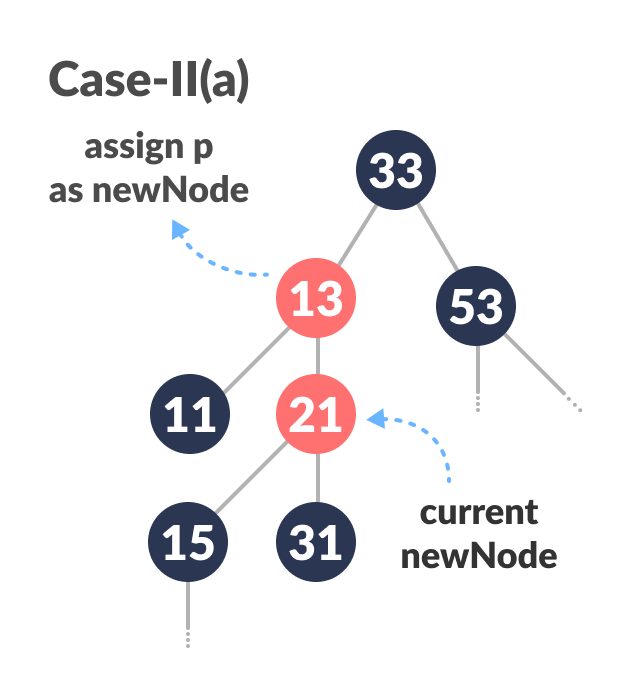Assigning parent of newNode as newNode
4. Left-Rotate `newNode`.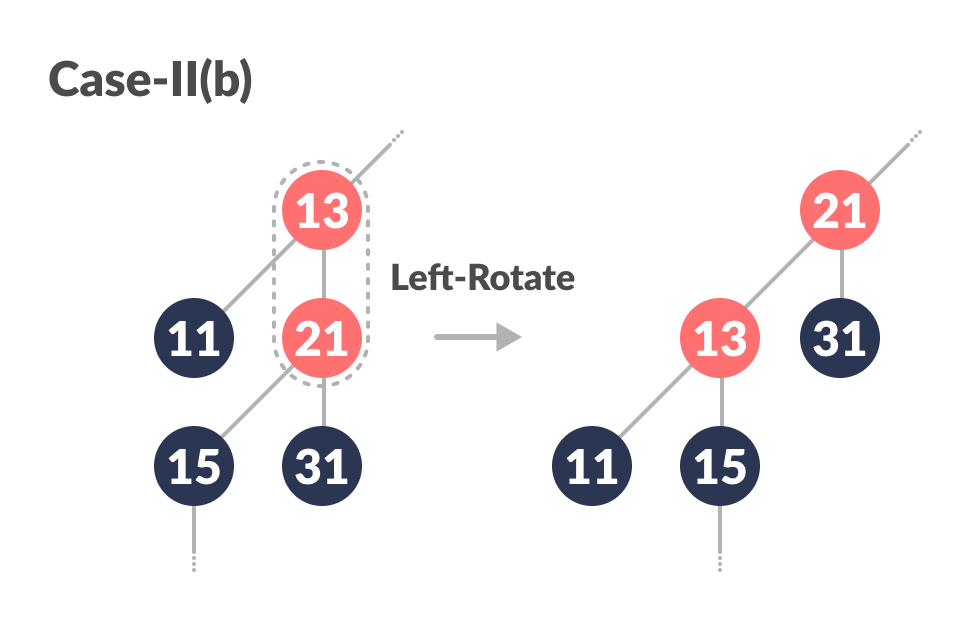Left Rotate

Case-III:

5. (Before moving on to this step, while loop is checked. If conditions are not satisfied, it the loop is broken.)
Set color of `p` as BLACK and color of `gP` as RED.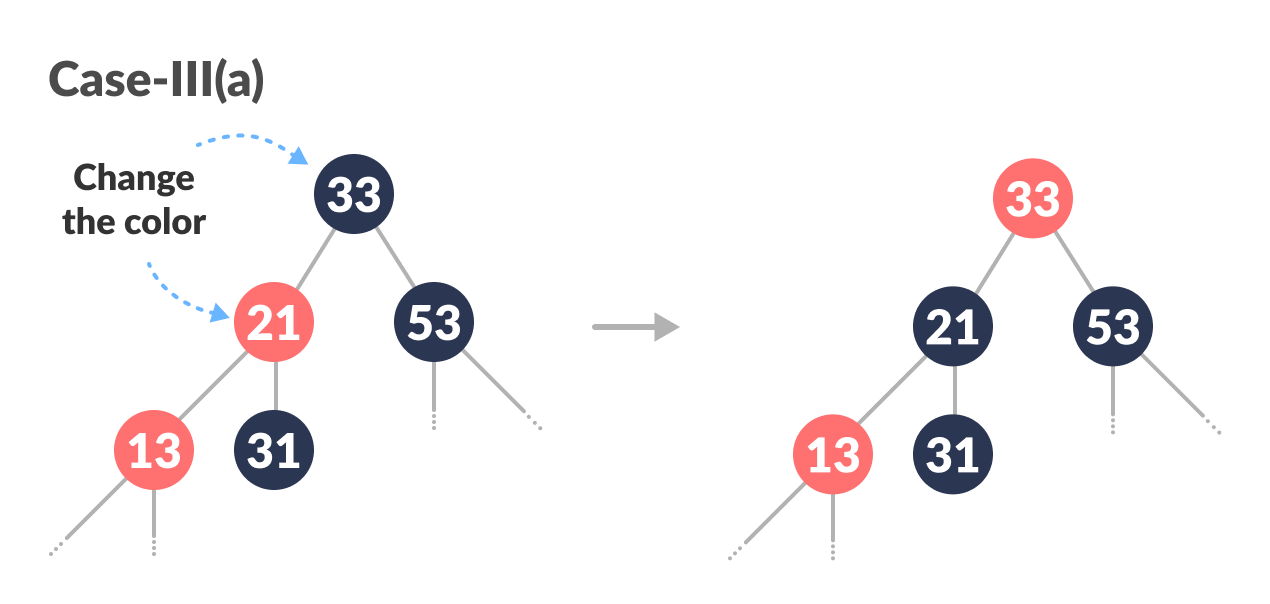Color change
6. Right-Rotate `gP`.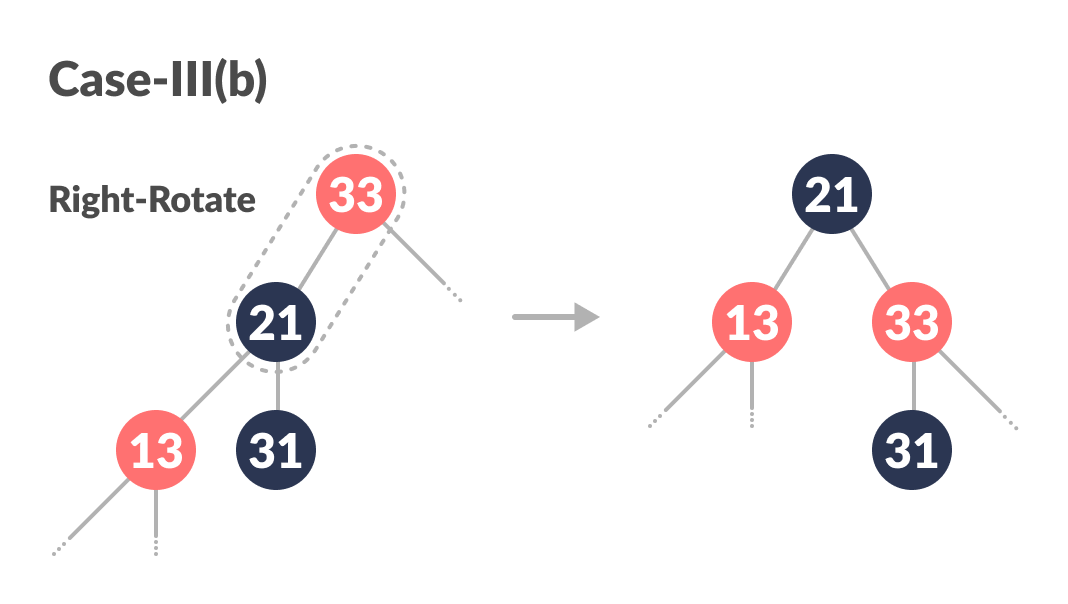Right Rotate
3. Else, do the following.
1. If the color of the left child of `gP` of `z` is RED, set the color of both the children of `gP` as BLACK and the color of `gP` as RED.
2. Assign `gP` to `newNode`.
3. Else if `newNode` is the left child of `p` then, assign `p` to `newNode` and Right-Rotate `newNode`.
4. Set color of `p` as BLACK and color of `gP` as RED.
5. Left-Rotate `gP`.
4. (This step is performed after coming out of the while loop.)
Set the root of the tree as BLACK.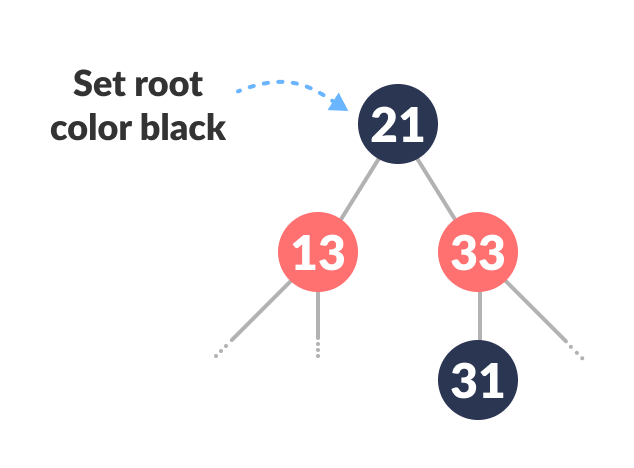Set root’s color black

The final tree look like this: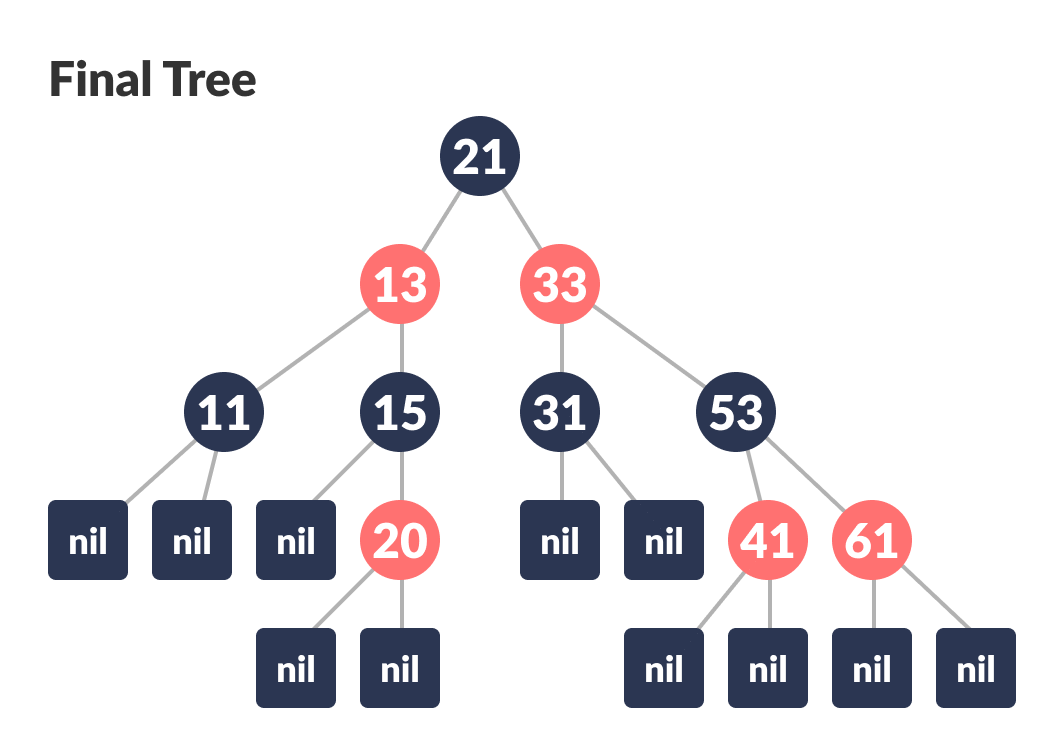Final tree

## Python Examples

``````/* Implementing Red-Black Tree in Python */

import sys

/* Node creation */
class Node():
def __init__(self, item):
self.item = item
self.parent = None
self.left = None
self.right = None
self.color = 1

class RedBlackTree():
def __init__(self):
self.TNULL = Node(0)
self.TNULL.color = 0
self.TNULL.left = None
self.TNULL.right = None
self.root = self.TNULL

/* Preorder */
def pre_order_helper(self, node):
if node != TNULL:
sys.stdout.write(node.item + " ")
self.pre_order_helper(node.left)
self.pre_order_helper(node.right)

/* Inorder */
def in_order_helper(self, node):
if node != TNULL:
self.in_order_helper(node.left)
sys.stdout.write(node.item + " ")
self.in_order_helper(node.right)

/* Postorder */
def post_order_helper(self, node):
if node != TNULL:
self.post_order_helper(node.left)
self.post_order_helper(node.right)
sys.stdout.write(node.item + " ")

/* Search the tree */
def search_tree_helper(self, node, key):
if node == TNULL or key == node.item:
return node

if key < node.item:
return self.search_tree_helper(node.left, key)
return self.search_tree_helper(node.right, key)

/* Balance the tree after insertion */
def fix_insert(self, k):
while k.parent.color == 1:
if k.parent == k.parent.parent.right:
u = k.parent.parent.left
if u.color == 1:
u.color = 0
k.parent.color = 0
k.parent.parent.color = 1
k = k.parent.parent
else:
if k == k.parent.left:
k = k.parent
self.right_rotate(k)
k.parent.color = 0
k.parent.parent.color = 1
self.left_rotate(k.parent.parent)
else:
u = k.parent.parent.right

if u.color == 1:
u.color = 0
k.parent.color = 0
k.parent.parent.color = 1
k = k.parent.parent
else:
if k == k.parent.right:
k = k.parent
self.left_rotate(k)
k.parent.color = 0
k.parent.parent.color = 1
self.right_rotate(k.parent.parent)
if k == self.root:
break
self.root.color = 0

/* Printing the tree */
def __print_helper(self, node, indent, last):
if node != self.TNULL:
sys.stdout.write(indent)
if last:
sys.stdout.write("R----")
indent += "     "
else:
sys.stdout.write("L----")
indent += "|    "

s_color = "RED" if node.color == 1 else "BLACK"
print(str(node.item) + "(" + s_color + ")")
self.__print_helper(node.left, indent, False)
self.__print_helper(node.right, indent, True)

def preorder(self):
self.pre_order_helper(self.root)

def inorder(self):
self.in_order_helper(self.root)

def postorder(self):
self.post_order_helper(self.root)

def searchTree(self, k):
return self.search_tree_helper(self.root, k)

def minimum(self, node):
while node.left != self.TNULL:
node = node.left
return node

def maximum(self, node):
while node.right != self.TNULL:
node = node.right
return node

def successor(self, x):
if x.right != self.TNULL:
return self.minimum(x.right)

y = x.parent
while y != self.TNULL and x == y.right:
x = y
y = y.parent
return y

def predecessor(self,  x):
if (x.left != self.TNULL):
return self.maximum(x.left)

y = x.parent
while y != self.TNULL and x == y.left:
x = y
y = y.parent

return y

def left_rotate(self, x):
y = x.right
x.right = y.left
if y.left != self.TNULL:
y.left.parent = x

y.parent = x.parent
if x.parent == None:
self.root = y
elif x == x.parent.left:
x.parent.left = y
else:
x.parent.right = y
y.left = x
x.parent = y

def right_rotate(self, x):
y = x.left
x.left = y.right
if y.right != self.TNULL:
y.right.parent = x

y.parent = x.parent
if x.parent == None:
self.root = y
elif x == x.parent.right:
x.parent.right = y
else:
x.parent.left = y
y.right = x
x.parent = y

def insert(self, key):
node = Node(key)
node.parent = None
node.item = key
node.left = self.TNULL
node.right = self.TNULL
node.color = 1

y = None
x = self.root

while x != self.TNULL:
y = x
if node.item < x.item:
x = x.left
else:
x = x.right

node.parent = y
if y == None:
self.root = node
elif node.item < y.item:
y.left = node
else:
y.right = node

if node.parent == None:
node.color = 0
return

if node.parent.parent == None:
return

self.fix_insert(node)

def get_root(self):
return self.root

def print_tree(self):
self.__print_helper(self.root, "", True)

if __name__ == "__main__":
bst = RedBlackTree()

bst.insert(55)
bst.insert(40)
bst.insert(65)
bst.insert(60)
bst.insert(75)
bst.insert(57)

bst.print_tree()``````

# Special 95% discount

## 2000+ Applied Machine Learning & Data Science Recipes

### Portfolio Projects for Aspiring Data Scientists: Tabular Text & Image Data Analytics as well as Time Series Forecasting in Python & R## Two Machine Learning Fields

There are two sides to machine learning:

• Practical Machine Learning:This is about querying databases, cleaning data, writing scripts to transform data and gluing algorithm and libraries together and writing custom code to squeeze reliable answers from data to satisfy difficult and ill defined questions. It’s the mess of reality.
• Theoretical Machine Learning: This is about math and abstraction and idealized scenarios and limits and beauty and informing what is possible. It is a whole lot neater and cleaner and removed from the mess of reality.

Data Science Resources: Data Science Recipes and Applied Machine Learning Recipes

Introduction to Applied Machine Learning & Data Science for Beginners, Business Analysts, Students, Researchers and Freelancers with Python & R Codes @ Western Australian Center for Applied Machine Learning & Data Science (WACAMLDS) !!!

Latest end-to-end Learn by Coding Recipes in Project-Based Learning:

Applied Statistics with R for Beginners and Business Professionals

Data Science and Machine Learning Projects in Python: Tabular Data Analytics

Data Science and Machine Learning Projects in R: Tabular Data Analytics

Python Machine Learning & Data Science Recipes: Learn by Coding

R Machine Learning & Data Science Recipes: Learn by Coding

Comparing Different Machine Learning Algorithms in Python for Classification (FREE)

`Disclaimer: The information and code presented within this recipe/tutorial is only for educational and coaching purposes for beginners and developers. Anyone can practice and apply the recipe/tutorial presented here, but the reader is taking full responsibility for his/her actions. The author (content curator) of this recipe (code / program) has made every effort to ensure the accuracy of the information was correct at time of publication. The author (content curator) does not assume and hereby disclaims any liability to any party for any loss, damage, or disruption caused by errors or omissions, whether such errors or omissions result from accident, negligence, or any other cause. The information presented here could also be found in public knowledge domains.  `